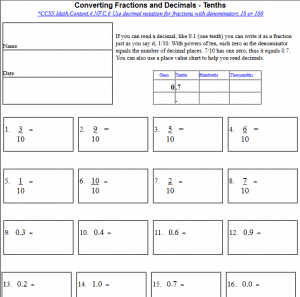# Converting Fractions and DecimalsConverting Fractions and Decimals (Tenths) - Free printable math common core worksheet that gives students the repetitive practice to change fractions into decimals and decimals into fraction.

Converting Fractions and Decimals (Hundredths) - Free printable math common core worksheet that gives students the repetitive practice to change fractions into decimals and decimals into fraction.

Converting Fractions and Decimals (Thousandths) - Free printable math common core worksheet that gives students the repetitive practice to change fractions into decimals and decimals into fraction.

CCSS.Math.Content.4.NF.C.6 Use decimal notation for fractions with denominators 10 or 100.
CCSS.Math.Content.5.NBT.A.3 Read, write, and compare decimals to thousandths.